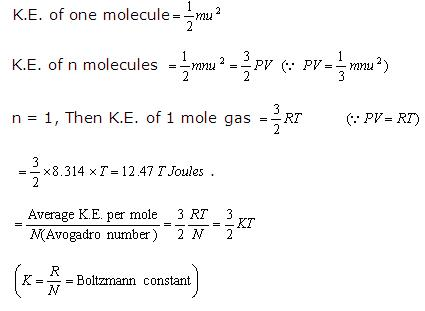1800-1023-196

+91-120-4616500

# Kinetic Theory of Gases

## Kinetic Theory of Gases

(1) Kinetic theory was developed by Bernoulli, Joule, Clausius, Maxwell and Boltzmann etc. and represents dynamic particle or microscopic model for different gases since it throws light on the behaviour of the particles (atoms and molecules) which constitute the gases and cannot be seen. Properties of gases which we studied earlier are part of macroscopic model.

(2) Postulates

(i) Every gas consists of a large number of small particles called molecules moving with very high velocities in all possible directions.

(ii) The volume of the individual molecule is negligible as compared to the total volume of the gas.

(iii) Gaseous molecules are perfectly elastic so that there is no net loss of kinetic energy due to their collisions.

(iv) The effect of gravity on the motion of the molecules is negligible.

(v) Gaseous molecules are considered as point masses because they do not posses potential energy. So the attractive and repulsive forces between the gas molecules are negligible.

(vi) The pressure of a gas is due to the continuous bombardment on the walls of the containing vessel.

(vii) At constant temperature the average K.E. of all gases is same.

(viii) The average K.E. of the gas molecules is directly proportional to the absolute temperature.

(3) Kinetic gas equation: On the basis of above postulates, the following gas equation was derived,

PV = (1/3) mnurms2

where, P = pressure exerted by the gas

V = volume of the gas

m = average mass of each molecule

n = number of molecules

u = root mean square (RMS) velocity of the gas.

(4) Calculation of kinetic energy

We know that,This equation shows that K.E. of translation of a gas depends only on the absolute temperature. This is known as Maxwell generalisation. Thus average K.E. ∝ T.

If T = 0K (i.e., -273.15°C) then, average K.E. = 0. Thus, absolute zero (0K) is the temperature at which molecular motion ceases.

## NEET & AIIMS Exam Sample Papers

 AIIMS SAMPLE PAPERS View More NEET SAMPLE PAPERS View More